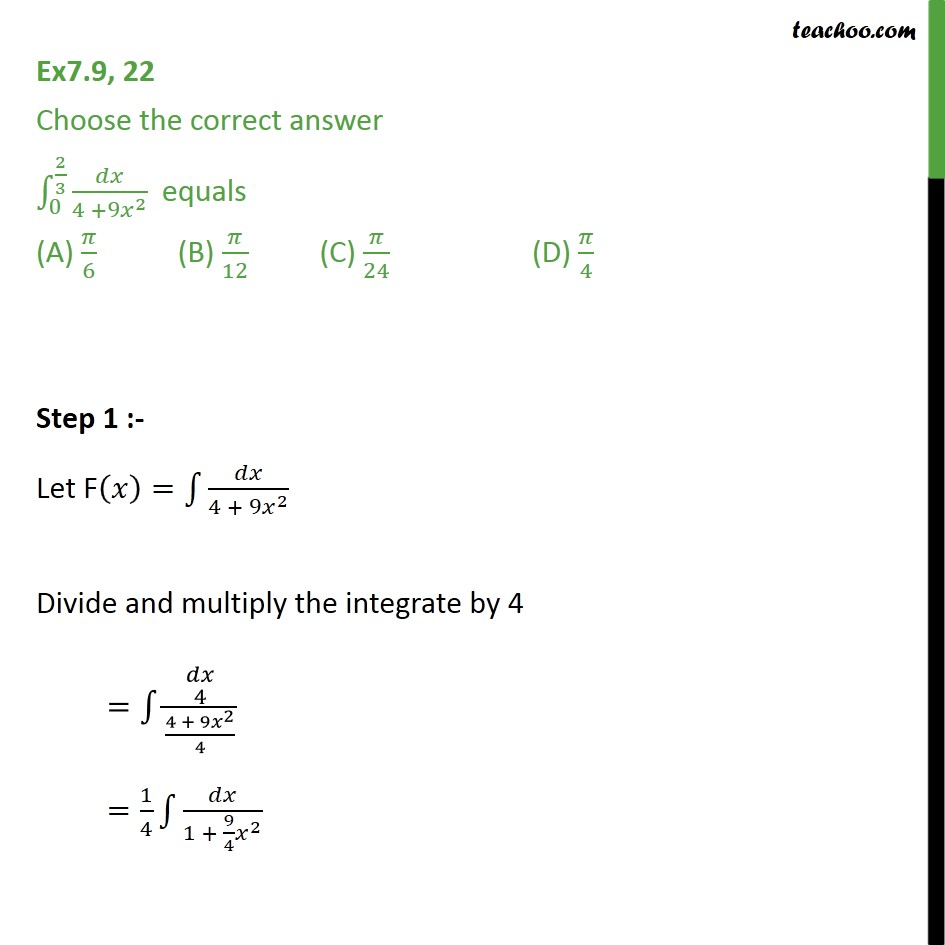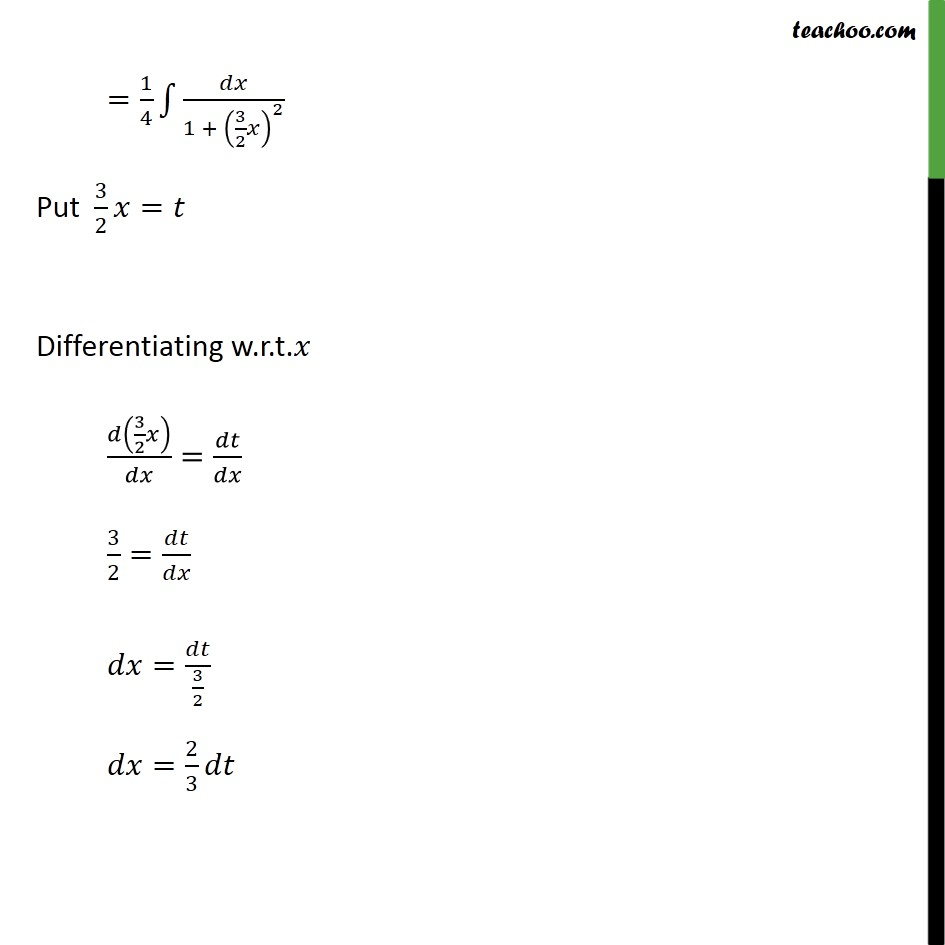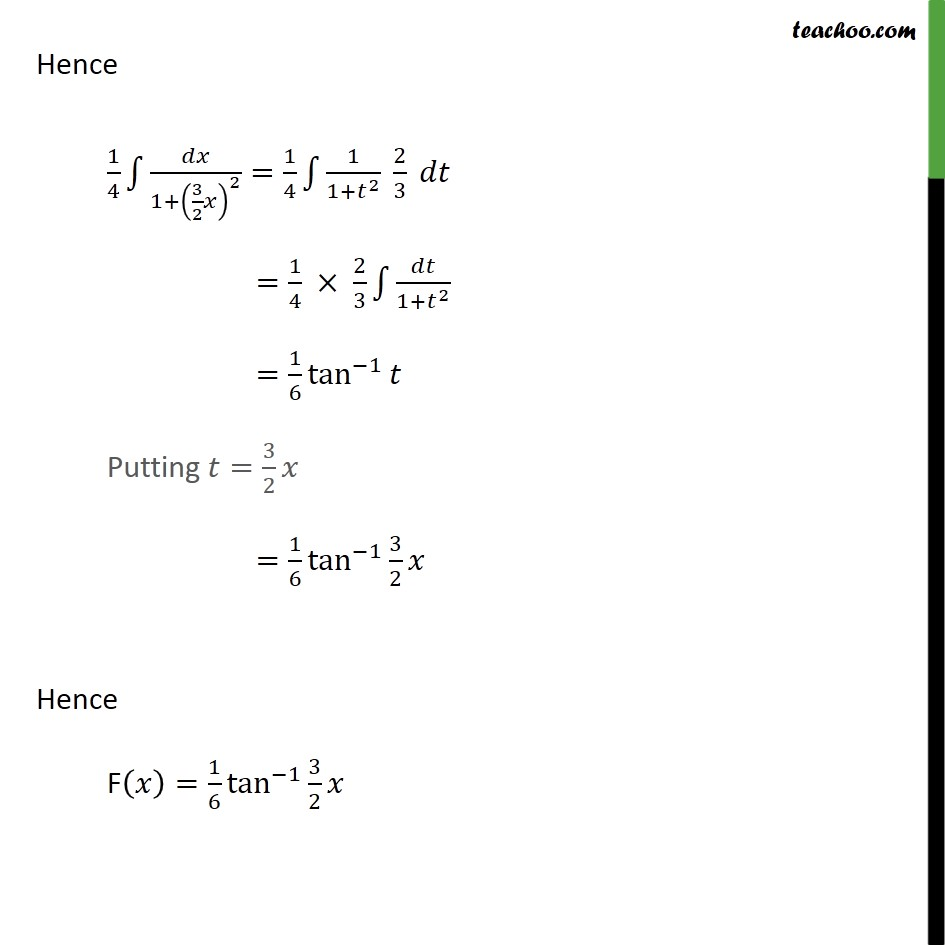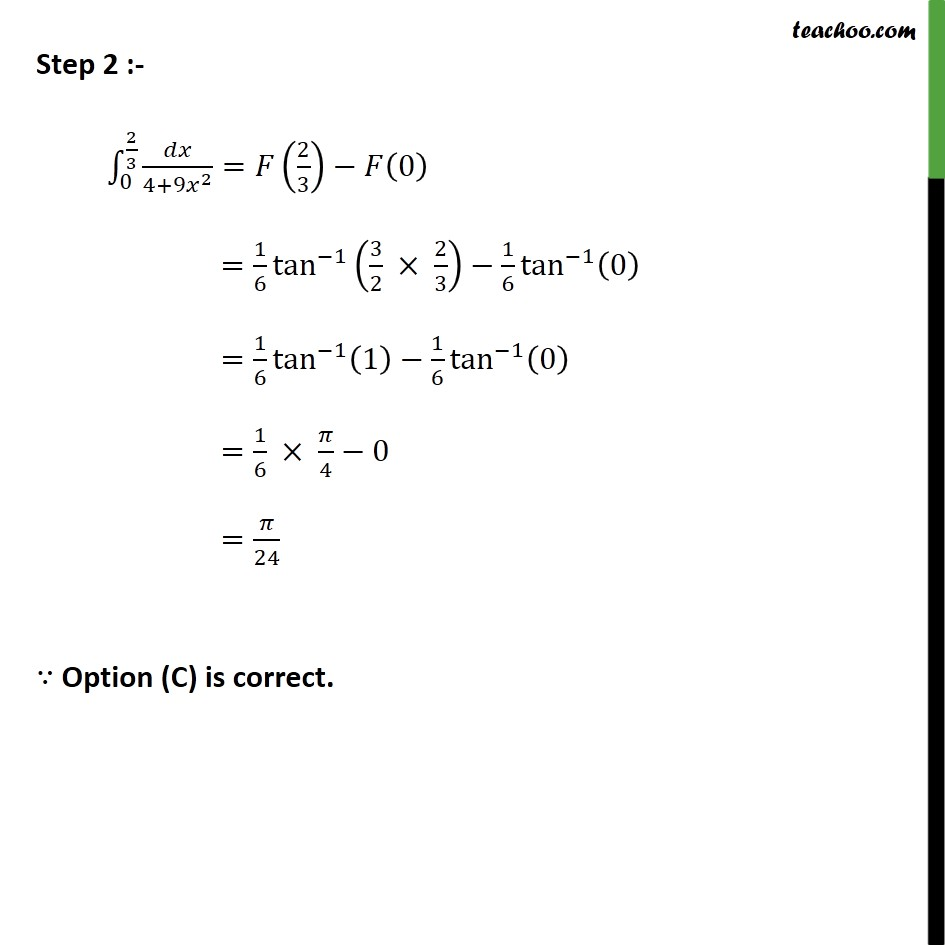Integration Full Chapter Explained - Integration Class 12 - Everything you need1. Chapter 7 Class 12 Integrals
2. Concept wise
3. Definite Integration - By Formulae

Transcript

Ex7.9, 22 Choose the correct answer ∫_0^(2/3)▒𝑑𝑥/(4 +9𝑥^2 ) equals (A) 𝜋/6 (B) 𝜋/12 (C) 𝜋/24 (D) 𝜋/4 Step 1 :- Let F(𝑥)=∫1▒𝑑𝑥/(4 + 9𝑥^2 ) Divide and multiply the integrate by 4 =∫1▒█(𝑑𝑥@4)/((4 + 9𝑥^2)/4) =1/4 ∫1▒𝑑𝑥/(1 + 9/4 𝑥^2 ) =1/4 ∫1▒𝑑𝑥/(1 + (3/2 𝑥)^2 ) Put 3/2 𝑥=𝑡 Differentiating w.r.t.𝑥 𝑑(3/2 𝑥)/𝑑𝑥=𝑑𝑡/𝑑𝑥 3/2=𝑑𝑡/𝑑𝑥 𝑑𝑥=𝑑𝑡/(3/2) 𝑑𝑥=2/3 𝑑𝑡 Hence 1/4 ∫1▒〖𝑑𝑥/(1+(3/2 𝑥)^2 )=1/4 ∫1▒〖1/(1+𝑡^2 ) 2/3〗 𝑑𝑡〗 =1/4 × 2/3 ∫1▒𝑑𝑡/(1+𝑡^2 ) =1/6 tan^(−1)⁡𝑡 Putting 𝑡=3/2 𝑥 =1/6 tan^(−1)⁡〖3/2 𝑥〗 Hence F(𝑥)=1/6 tan^(−1)⁡〖3/2 𝑥〗 Step 2 :- ∫_0^(2/3)▒〖𝑑𝑥/(4+9𝑥^2 )=𝐹(2/3)−𝐹(0) 〗 =1/6 tan^(−1)⁡〖(3/2 × 2/3)−1/6 tan^(−1)⁡(0) 〗 =1/6 tan^(−1)⁡〖(1)−1/6 tan^(−1)⁡(0) 〗 =1/6 × 𝜋/4−0 =𝜋/24 ∵ Option (C) is correct.

Definite Integration - By Formulae

About the AuthorDavneet Singh
Davneet Singh is a graduate from Indian Institute of Technology, Kanpur. He has been teaching from the past 9 years. He provides courses for Maths and Science at Teachoo.